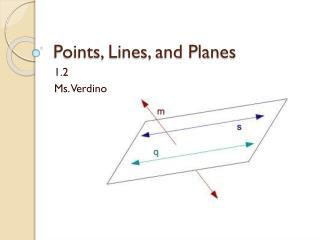DownloadDownload PresentationPoints, Lines, and Planes

# Points, Lines, and Planes

Download Presentation## Points, Lines, and Planes

- - - - - - - - - - - - - - - - - - - - - - - - - - - E N D - - - - - - - - - - - - - - - - - - - - - - - - - - -
##### Presentation Transcript

1. Points, Lines, and Planes 1.2 Ms. Verdino

2. What will we be learning today? SPI 3108.1.4: Use definitions, basic postulates, and theorems about points, lines, angles, and planes to write/complete proofs and/or to solve problems. Objective To understand basic terms and postulates of geometry.

3. Euclid 300 BC • Born in Greece • Wrote the book the “Elements” • Known as the Father of geometry • Developed 5 postulates • 3 undefined definitions

4. The undefined terms • Point: Indicates a location and has no size. • Line: Represented by a straight path that extends in two opposite • directions without end and has no thickness. A line also contains • infinitely many points. • Plane: Represented by a flat surface that extends without end and has • no thickness. A plan contains infinitely many lines.

5. Collinear points: Points on the same line. • Coplanar points: Points and lines that lie in the same plane. What are two other ways to rename CE ? What are two other ways to rename plane X ? What are the names of three collinear points? What are the names of four coplanar points?

6. Your turn! What are two other ways to name plane V? Name three collinear points. Are points R, N, M, and X coplanar? Name four coplanar points.

7. Space: The set of all points in three dimensions. • Segment: Part of a line that consists of two endpoints • and all points between them. • Ray: Part of a line that consists of one endpoint and all the points of • The line on one side of the endpoints. • Opposite rays: Two rays that share the same endpoint and form a line.

8. Name four segments on the figure above. Name four rays on the figure above. Name 2 pairs of opposite rays on the figure above.

9. Your turn! What are the names of the segments in the figure above? What are the names of the rays in the figure above? Which of the rays in part 2 are opposite rays?

10. Postulates and Axioms • Postulate/Axiom : An accepted statement of fact. • : Building blocks of the logical system in geometry. • Theorem: A general proposition not self-evident but proved by a chain • of reasoning; a truth established by means of accepted truths Postulate 1-1 Through any two points there is exactly one line.

11. Postulate 1-2 When you have two or more geometric figures, the intersection is the set of points that figures have in common. If two distinct lines intersect, then they intersect in exactly one point.

12. Postulate 1-3 If two distinct planes intersect, then they intersect in exactly one line

13. Postulate 1-4 Through any three noncollinear points a there is exactly one plane.

14. Each surface in the figure on the right represents a plane. What is the intersection of plane ADC and plane DRQ? What plane contains points B, C, and Q?

15. Your turn! What is the intersection of plane ABC and EFB? What plane contains points E, F, and C?